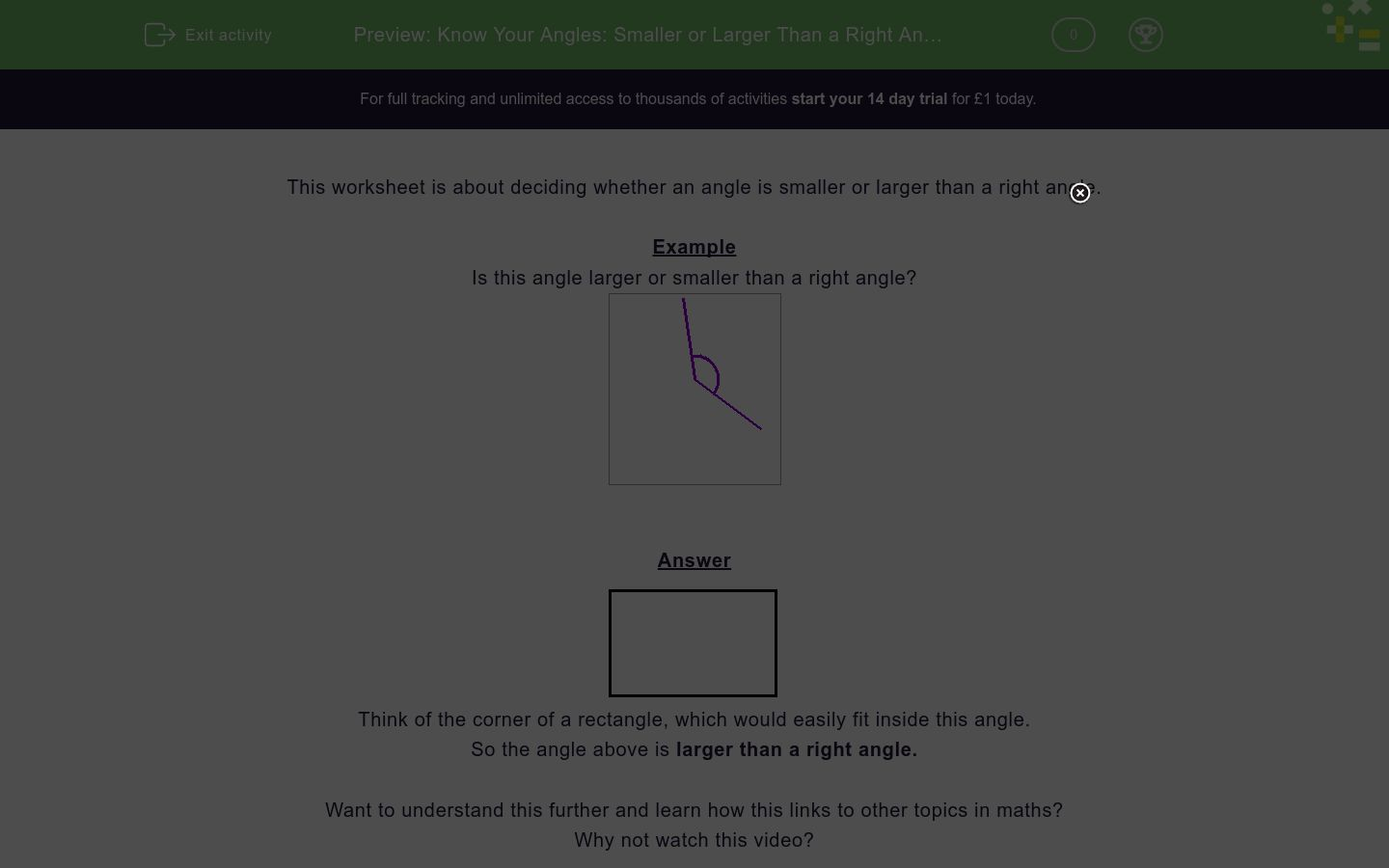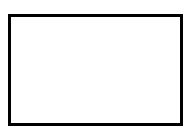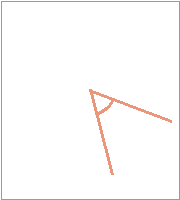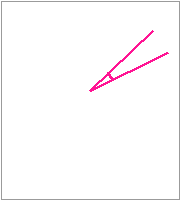# Know Your Angles: Smaller or Larger Than a Right Angle?

In this worksheet, students decide whether an angle is larger or smaller than a right angle.Key stage:  KS 2

Curriculum topic:   Geometry: Properties of Shapes

Curriculum subtopic:   Recognise Angles

Difficulty level:### QUESTION 1 of 10

This worksheet is about deciding whether an angle is smaller or larger than a right angle.

Example

Is this angle larger or smaller than a right angle?Think of the corner of a rectangle, which would easily fit inside this angle.

So the angle above is larger than a right angle.

Want to understand this further and learn how this links to other topics in maths?
Why not watch this video?

Is this angle larger or smaller than a right angle?larger than a right angle

smaller than a right angle

Is this angle larger or smaller than a right angle?larger than a right angle

smaller than a right angle

Is this angle larger or smaller than a right angle?larger than a right angle

smaller than a right angle

Is this angle larger or smaller than a right angle?larger than a right angle

smaller than a right angle

Is this angle larger or smaller than a right angle?larger than a right angle

smaller than a right angle

Is this angle larger or smaller than a right angle?larger than a right angle

smaller than a right angle

Is this angle larger or smaller than a right angle?larger than a right angle

smaller than a right angle

Is this angle larger or smaller than a right angle?larger than a right angle

smaller than a right angle

Is this angle larger or smaller than a right angle?larger than a right angle

smaller than a right angle

Is this angle larger or smaller than a right angle?larger than a right angle

smaller than a right angle

• Question 1

Is this angle larger or smaller than a right angle?larger than a right angle
• Question 2

Is this angle larger or smaller than a right angle?smaller than a right angle
• Question 3

Is this angle larger or smaller than a right angle?smaller than a right angle
• Question 4

Is this angle larger or smaller than a right angle?larger than a right angle
• Question 5

Is this angle larger or smaller than a right angle?larger than a right angle
• Question 6

Is this angle larger or smaller than a right angle?larger than a right angle
• Question 7

Is this angle larger or smaller than a right angle?smaller than a right angle
• Question 8

Is this angle larger or smaller than a right angle?smaller than a right angle
• Question 9

Is this angle larger or smaller than a right angle?smaller than a right angle
• Question 10

Is this angle larger or smaller than a right angle?larger than a right angle
---- OR ----

Sign up for a £1 trial so you can track and measure your child's progress on this activity.

### What is EdPlace?

We're your National Curriculum aligned online education content provider helping each child succeed in English, maths and science from year 1 to GCSE. With an EdPlace account you’ll be able to track and measure progress, helping each child achieve their best. We build confidence and attainment by personalising each child’s learning at a level that suits them.

Get started